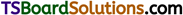# TS Inter 1st Year Chemistry Notes Chapter 7 Chemical Equilibrium and Acids-Bases

Here students can locate TS Inter 1st Year Chemistry Notes 7th Lesson Chemical Equilibrium and Acids-Bases to prepare for their exam.

## TS Inter 1st Year Chemistry Notes 7th Lesson Chemical Equilibrium and Acids-Bases

→ The mixture of reactants and products in the equilibrium state is called an equilibrium mixture.

→ Reactions which proceed in one direction only are called irreversible reactions.

→ Reactions which proceed from reactants to products and also from products to reactants, simultaneously are called reversible reactions.

→ The state of a reversible reaction at which the rate of forward reaction is equal to the rate of backward reaction is called Equilibrium.

→ Chemical equilibrium is dynamic.

→ Increased concentration of reactants shifts the equilibrium position to products side and increased concentration of products shifts the equilibrium position to reactants side.

→ High temperature favours Exothermic reaction and low temperature favours Endothermic reaction.

→ A catalyst has no influence on the position of equilibrium. The catalyst is used to establish the equilibrium quickly.→ For a general reaction aA + bB ⇌ cC + dD
Kc = $$\frac{[\mathrm{C}]^{\mathrm{c}}[\mathrm{D}]^{\mathrm{d}}}{[\mathrm{A}]^{\mathrm{a}}[\mathrm{B}]^{\mathrm{b}}}$$; Kp = $$\frac{P_C^c P_D^d}{P_A^a P_B^b}$$
Kp = Kc(RT)Δn; Δn = (c + d) – (a + b)

→ For, H2 + I2 ⇌ 2HI; Kp = Kc
For, N2 + 3H2 ⇌ 2NH3; Kp < Kc (∵ Δn = -2)
For, PCl5 ⇌ PCl3 + Cl2; Kp > Kc (∵ Δn = 1)

→ Le Chatelier principle states that When a system at equilibrium was subjected to stress, the system shifts in such a way so as to undo the effect of the change.

→ According to Le Chatelier principle, High pressure and low temperature are favourable in Haber’s process and in Con-tact process.

→ Substances are classified into acids, bases and salts depending on their chemical behaviour and properties.

→ According to Bronsted theory proton donor is an acid and proton acceptor is a base.

→ An acid and a base which differ by a single proton are called conjugate acid-base pairs.

→ Leveling by water of all strong acids to the strength of H3O+ and all strong bases to the strength of OH ions is called leveling effect of water.

→ According to Lewis electron pair acceptor is an acid and electron pair donor is a base.

→ The product of hydrogen ion (H+) and hydroxyl ion (OH) in pure water or in any aqueous solution is called ionic product of water.

→ The negative value of the logarithm of the hydrogen ion concentration is called pH.
A solution which resists the change in its pH value on dilution or on addition of small amounts of acid or base is called buffer solution.

→ The number of moles of acid or base requi-red to be added to the one litre of a buffer solution to register a change of one unit in the pH is called buffer capacity (Φ).

→ The substance which exhibits one colour in acidic medium and another colour in alkaline medium is known as acid-base indicator.→ The interaction of anion or cation or both of a salt with water to form basic or acidic or neutral solution is known as salt hydrolysis.

→ The aqueous solutions of salts of weak acid and strong base are basic. The aqueous solutions of salts of weak base and strong acid are acidic.

→ The product of the concentrations of the cation and the anion in a saturated solution of a salt at room temperature is called solubility product (Ksp)
Ksp = [Mn+][An-]

→ Relation between solubility product (Ksp) and solubility (s) is, S = $$\left[\frac{K_{S p}}{x^x y^y}\right]^{y_{x+y}}$$

→ The solubility of an electrolyte in water decreases on addition of an electrolyte which has one ion common with the electrolyte, is called common ion effect.

→ Common ion effect principle is used in the systematic qualitative analysis of cations.